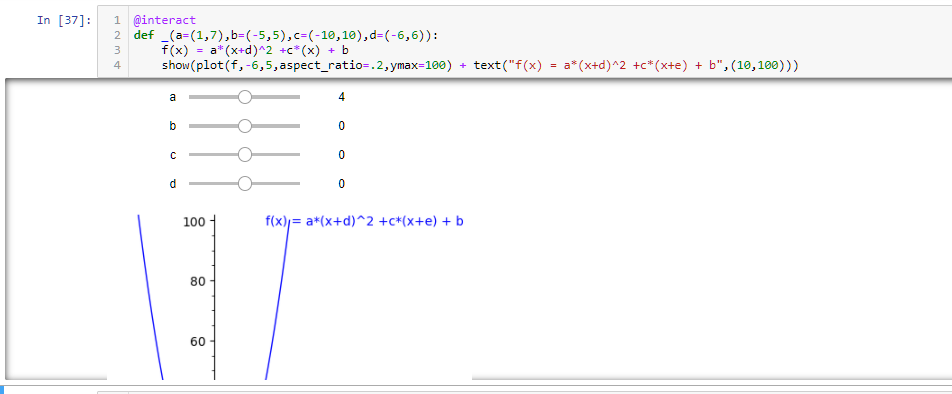# lengthen interact window or move controls to side

Is there any way I can lengthen the Interact window or move the controls to the side? When I have more than two controls it cuts off the function plot at the bottom so it's hard to see the effect.

@interact
def _(a=(1,7),b=(-5,5),c=(-10,10),d=(-6,6)):
f(x) = a*(x+d)^2 +c*(x) + b
show(plot(f,-6,5,aspect_ratio=.2,ymax=100) + text("f(x) = a*(x+d)^2 +c*(x+e) + b",(10,100)))edit retag close merge delete

Sort by » oldest newest most voted

Found the answer: Cell > Current Outputs > Toggle Scrolling so you get the full height of the output window.

more